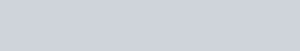# Rod-clocks in a frame of reference

Space is the three-dimensional domain in which motion occurs. Time is a one-dimensional domain in which a reference uniform motion occurs.

The extent of a motion is measured either as a length or a duration by a rod-clock. A rod-clock is a linear rod combined with a linear clock, like this:The pointer moves in uniform motion along the rod at a rate set by convention and points to both sides of the rod. The length side is marked in units of length; the duration side is marked in units of duration.

When rods are attached end-to-end, the pointer keeps going, like a monorail train. A stop point can be set at an event to be measured, as with a count-up timer.

A frame of reference is formed from a lattice of rod-clocks. It forms a kind of monorail system for measuring the locations of points and chronations of instants.

The rod measures the position of a point of a motion adjacent to the rod-clock. The position of a point is its location. The clock pointer measures the position of an instant of a motion adjacent to the rod-clock. The position of an instant is its chronation.

A time frame is a one-dimensional series of rod-clocks attached to another rod-clock at each end. The current location of a time frame pointer is called the distance. The current chronation of a time frame pointer is called the date-time.

A space frame of a body is a frame of reference at rest relative to it. Chorocosm is length space with time. Chronocosm is duration space with distance.

The location of an event is measured where a rod meets the event. A displacement is the vector from one location to another. The magnitude of the displacement is the distance between the locations. Length is a distance or distances or an infinite series of distances.

The chronation of an event is measured when a clock pointer reaches the event. A dischronment is the vector from one chronation to another. The magnitude of the distimement is the distime between the locations. Duration is a distime or distimes or an infinite series of distimes.